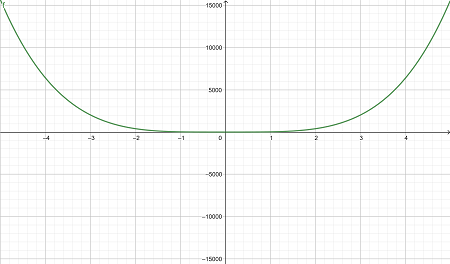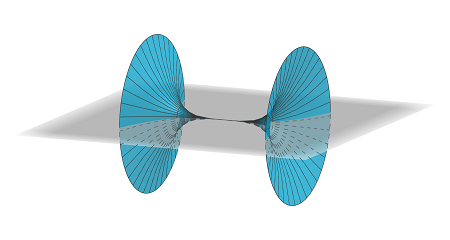# Find parametric equations for the surface obtained by rotating the curve y = 25 x^4 - x^2, - 5...

## Question:

Find parametric equations for the surface obtained by rotating the curve {eq}\displaystyle y = 25 x^4 - x^2,\ - 5 \le x \le 5 {/eq} about the x-axis, and use them to graph the surface.

## Parametric Surface:

Recall that a parametric curve can be defined by a set of parametric equations {eq}x(t),\, y(t),\, z(t) {/eq}. A parametric surface is defined by {eq}x(u,v),\, y(u,v),\, z(u,v) {/eq} where {eq}x,\, y {/eq} and {eq}z {/eq} are functions of two parameters.

We also recall that if we fix a point {eq}(x_0,y_0) {/eq} in the {eq}x {/eq} by plane and rotate around the {eq}x {/eq}-axis, then we get a circle with parametric equation {eq}x=x_0,\, y=y_0\sin u,\, z=y_0\cos u {/eq}. We can extend this to say if we rotate the parametric curve {eq}x(t),\, y(t) {/eq} about the {eq}x {/eq}-axis, then we get a parametric surface

{eq}x=x(t),\, y=y(t)\sin u,\, z=y(t)\cos u {/eq}.

The curve {eq}y=25x^4-x^2,\, -5\le x\le 5 {/eq} can be written as the parametric equations

{eq}x=t,\, y=25t^4-t^2,\, -5\leq t\leq 5 {/eq}. If we rotate this about the {eq}x {/eq}-axis, we get a surface with parametric equations

{eq}x=t,\, y=(25t^4-t^2)\sin u,\, z=(25t^4-t^2)\cos u,\, -5\leq t\leq 5,\, 0\leq u\leq 2\pi {/eq}.

A sketch of {eq}y=25x^4-x^2 {/eq} is shown belowRotating this about the {eq}x {/eq}-axis we get the following